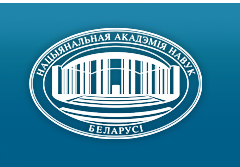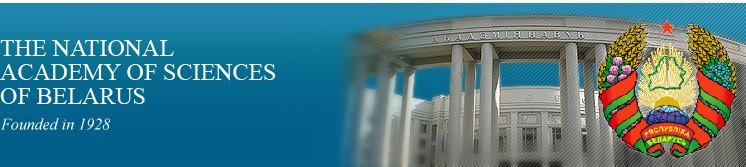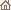Home News About Academy Academy Activities Academy Membership Organizations Publications Conferences Contacts Site Map

# Proc. of the Academy of Sciences of Belarus, Ser. Phys.-Math. Sci., 1996, No.1/ Publications / Scientific Journals

Proc. of the Academy of Sciences of Belarus, Ser. Phys.-Math. Sci., 1996, No.1Proceedings of the Academy of Sciences of Belarus (Vestsi Akademii Navuk Belarusi) SERIES OF PHYSICAL-MATHEMATICAL SCIENCESPublished by The Belaruskaya Navuka Publishing House, Minsk, Republic of Belarus

### Number 1, 1996## CONTENTS

### MATHEMATICS

Grudo E.I.
On quasi-normal form of matrix of several variables. pp. 5--7

Summary: The method of reducing quadratic continuous matrix of several variables to quasinormal form is represented.

Zhavrid N.S., Yanovich L.A.
Quadratic interpolation of functionals in the spaces of the continuously differentiable functions of one and two variables. pp. 8--15

Summary: The quadratic interpolation formulas for functionals in the spaces of the continuously differentiable functions of one and two variables are derived.

Bobkov V.V.
On an approach to the construction of numerical methods for systems of ordinary differential equations. pp. 15--19

Summary: A new approach to the construction of numerical methods for initial value problems for autonomous systems of ordinary differential equations (including stiff systems) is proposed. This approach is oriented to the procedure of linearization of the nonlinear system at each step of integration. The essence of the technique is the use of polynomial approximation of the matrix exponential function with respect to the matrix argument. It is important that the stability property holds for any degree of the polynomial and for any positive value of the discretization step.

Gorbuzov V.N.
On integrals and last multipliers of multidimensional differential systems with symmetries. pp. 20--24

Summary: Construction of the last multipliers and the first integrals of the system (I) on the base of linear differential operators is carried out.

Brui I.N.
Trigonometric rows of Lp(T) classes and their regular means. pp. 24--30

Summary: For the case when r(n)m = max(1 - m(n + 1)-1; 0) theorem has been proved by W.H. and G.C. Young (1913).

Dubrovsky V.V.
Regulirized traces of self-adjoint operators. pp. 30--33

Summary: Author proved the regularization of traces for discrete self-adjoint operators in sense of row Taylor.

Avdashkova L.P., Kamornikov S.F.
On normalizers of F-reachable subgroups. pp. 33--35

Summary: For S-closed formation F it is shown that normalizer of each F-reachable subgroup of finite group G contains F-residual of socle of this group.

### PHYSICS

Redkov V.M.
Existence of a spinor apace geometry and the concept of intrinsic fermion parity. pp. 36--42

Summary: Some traditional approaches concerning the intrinsic fermion parity suffer from grave shortcomings. Analysing the fermion parity in the context of utilizing a spinor space geometry is proposed in this article. In such a space model, the 2 ¶-rotation does not amount to the identical transformation I, and the whole problem of intrinsic fermion parities is to be solved by a classification of non-equivalent representations of the geometrical symmetry group. Extended according to E. Cartan the 2-dimension unitary group {SU(2) + J) = SU~(2) allows two types of non-relativistic fermions. The 4-dimension extended linear spinor group SL(2.C), covering the Lorentz group L+-|| which contains P- and T-reflections, admits two types of relativistic fermions too. The concept of boson intrinsic parity is to be seriously revised in the spinor geometry framework.

Sergeenko M.N.
Isotropic oscillator in quasi-classical approach. pp. 43--49

Summary: The question on the application of the quasi-classical approach for solution of multidimensional problems in quantum mechanics is investigated. The method of asymptotical quantization based on the solution of quasi-classical wave equation obtained by the correspondence principle and adiabatic one with classical Hamilton--Jacobi equation is proposed. The problem of isotropic oscillator is solved to demonstrate the efficiency of proposed quasi-classical method. Obtained after division of the variables the one-dimensional equations (radial and angular ones) are solved by the proposed method that results in the well-known exact eigenvalues of isotropic oscillator and correspondent eigenfunctions of standing wave type.

Revinsky A.F.
Calculation from the first principles of potentials of electron intervalley scattering on phonons. pp. 49--52

Summary: The deformation potential for electron scattering between the equivalently oriented valleys (g-type intervalley scattering) is calculated for silicon using the density functional theory.

Tsvetkov I.V.
Calculation of functional integrals by the operator method. II. pp. 52--57

Summary: In the framework of the Operator Method of the uniformly fitted estimation of functional integrals a simple and general procedure for calculating the terms of the expanding the Exponential is derived. With reference to the problem on non-relativistic anharmonic oscillator a second-order correction to the ground energy is obtained and compared with the tabulated numerical values. The agreement is excellent.

Dostanko A.P., Shiripov V.Ya., Fedosenko G.N., Yemelianov V.A., Maryshev S.P.
Analysis of diamond-like carbon film formation from laser plasma. pp. 58--61

Summary: The characterization of laser plasma during diamond-like carbon film formation by pulsed laser evaporation is reported.

Bogatyrev Yu.V.
Current annealing of irradiated CMOS integrated structures. pp. 61--65

Summary: Physical aspects and the current annealing method of irradiated CMOS integrated structures have been considered.

Sokolovsky T.D., Ryzhkovsky V.M.
Calculation of lattice and magnetic heat capacity of Mn2Sb compounds. pp. 65--69

Summary: Calculations of the bond energy, the dispersion relations and the specific heat of the manganese antimonide with account of anharmonicity at the temperature 0 -- 850 K are fulfilled. The contribution of the electronic and magnetic specific heat is also calculated.

Akimov A.I., Yakimovich V.N., Rubtsov V.A., Savchuk G.K.
Production and analysis of new compound in the Ba -- Cu -- O system. pp. 69--73

Summary: An unknown compound with the chemical formula BaCuO3-x was shown to be formed in the Ba -- Cu -- O system under oxygen pressure above 1 MPa and the temperature of 1000 °C. Parameters of this compound crystal lattice are -- a = 11.43 A°, b = 12.86 A°, c = 14.58 A°. The compound is decomposed at heating up to 1170 K with formation of BaCuO2 and it loses some part of oxygen at heating up to 600 °C in the air without any crystal structure change.

Margolin L.N., Gontarev V.F. Dielectric and polarization properties of triglycine sulfate doped by L-valine. pp. 74--77

Summary: The experimental investigation of dielectric and polarization properties of triglycine sulfate crystals doped with L-valine was carried out. Doping of triglycine sulfate crystals with L-valine reduces twice threshold, saturated and coercitive fields.

Sirota N.N., Vitkina Ts.Z., Shostak L.M.
Determination of kinetic parameters of cellulose thermal destruction. pp. 77--83

Summary: Investigation of thermal destruction processes of microcrystalline cellulose has been carried out by a thermogravimetry method. The kinetic parameters of thermal destruction reactions of microcrystalline cellulose have been determined from thermal analysis data on the basis of the method developed.

Batishche S.A., Mostovnikov V.A., Nikitchenko V.M., Tarkovsky V.V.
Investigation of generation characteristics of new coumarin dyes under flashlamp pumping. pp. 83--87

Summary: New coumarin dyes generating in the spectral range of 420 -- 657 nm with energy efficiency up to 1% and photochemical stability 3 -- 5 times as high as for Rodamin G are investigated.

Khilo P.A.
Generation of the second optical harmonic under its collinear diffraction by an ultrasound. pp. 88--90

Summary: The influence of the sediment from Bragg synchronism at acoustooptic interaction on the SHG area phases being in agreement has been studied. Providing modified conditions of the phase synchronism the estimation of transformation efficiency has been done.

### INFORMATICS

Kolyada A.A., Revinsky V.V., Selyaninov M.. Yu., Chernyavsky A.F.
Application of minimal redundant modular encoding for fast multiplication of complex numbers in the systems of digital signal processing. pp. 91--97

Summary: A novel method of multiplication of Gaussian's numbers to fractional complex constants is designed. It is based on use of minimum redundant modular number systems (MNS) and strategy of symmetrization of error's range. The proposed method allows double decrease of code's redundancy in comparison with classical MNS just when the execution time of operation is minimized.

Likhoded N.A.
A method for constructing algorithm parallel forms for reduce-size array processor implementation. pp. 97--104

Summary: A formalized method for the construction of parallel forms of algorithms for implementation on reduce-size array processors is proposed (reduce-size array processors are two-dimensional, the width of the arrays is fixed by preassigned number of processor elements). The method includes the partition of the dependence graph of algorithm and space-time mapping of each part into computation graph. Proposed approach leads to array processors without global interconnections, the local memory of the processor elements is independent of the number of parts.

Dailyudenko V.F., Krot A.M.
Nervous pulse propagation modeling on the basis of digital filtration methods. pp. 104--111

Summary: The article deals with the digital filtering method for kinetic model analysis of autowave processes. It is shown that the numerical model of nervous impulse propagation may be implemented by means of two linear filters construction. The main advantages of proposed method are the reduction of calculation complexity and increase of algorithm robustness.

Kravtsov M.K.
On unsolvability of discrete optimization vector problems by linear convolution algorithms. pp. 111--116

Summary: It is proved that vector problems on graphs and a variety of boolean programming problems cannot be solved by means of linear convolution algorithms.

### SHORT COMMUNICATIONS

Sheshko M.A.
Spectral ratios for singular integrals with Cauchy nucleus along the real axis. pp. 117--119

Summary: The so-called "Spectral relations" for singular integrals with Cauchy kernel were revealed, the line of integration being the whole material axis and also their analogs for regular integrals.

### ANNOTATIONS OF THE DEPOSITED ARTICLES

Basik V.A., Gladun O.M., Strezh A.I.
On composition of methods of complete reduction and matrix run. p.120

Strezh A.I.
On a variant of multigrid method of solving differential equations in combination with indistinct run. pp. 120--121

Vorobiev G.N.
On conjugation of n-subgroups. p.121

Suprun V.P., Lichko Yu.V.
Resolution of elementary and monotone symmetric Bool functions into monotonically polarized Reed--Muller polynomials. pp. 121--122/ Publications / Scientific Journals / Back to Top of Page

Designed and maintained by Dr. Nikolai N. Kostyukovich. Last updated: January 31, 1999
Created with assistance of Dr. Ignatii I. Korsak
Copyright © 1996-1999 The National Academy of Sciences of Belarus
Copyright © 1996 The Belaruskaya Navuka Publishing House